一篇文章教你用 11 行 Python 代码实现神经网络

Very simple Neural Network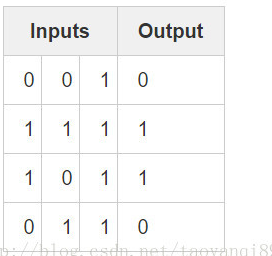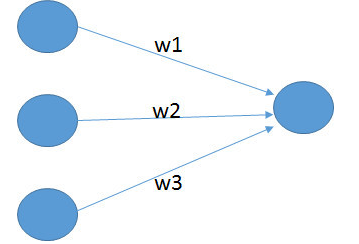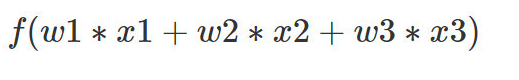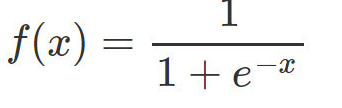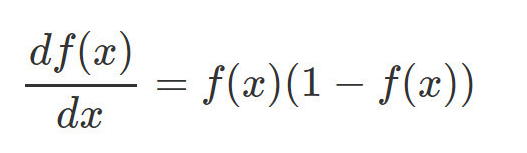1. 前向传播求损失
2. 反向传播更新w

import numpy as np
# sigmoid function
# deriv=ture 是求的是导数
def nonlin(x,deriv=False):
if(deriv==True):
return x*(1-x)
return 1/(1+np.exp(-x))
# input dataset
X = np.array([ [0,0,1],
[1,1,1],
[1,0,1],
[0,1,1] ])
# output dataset
y = np.array([[0,1,1,0]]).T
# seed random numbers to make calculation
np.random.seed(1)
# initialize weights randomly with mean 0
syn0 = 2*np.random.random((3,1)) - 1
# 迭代次数
for iter in xrange(10000):
# forward propagation
# l0也就是输入层
l0 = X
l1 = nonlin(np.dot(l0,syn0))
# how much did we miss?
l1_error = y - l1
# multiply how much we missed by the
# slope of the sigmoid at the values in l1
l1_delta = l1_error * nonlin(l1,True)
# update weights
syn0 += np.dot(l0.T,l1_delta)
print "Output After Training:"
print l1

l1_error = y - l1 同样为一个 4*1 的向量。

# slope of the sigmoid at the values in l1
#nonlin(l1,True),这里是对sigmoid求导
#前向计算，反向求导
l1_delta = l1_error * nonlin(l1,True)
# update weights
syn0 += np.dot(l0.T,l1_delta)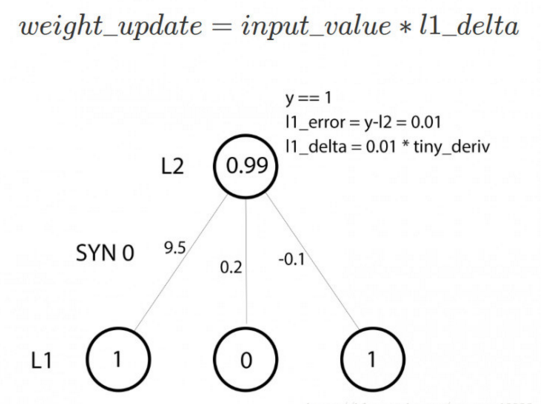Output After Training:
Output After Training:[[ 0.00966449]
[ 0.99211957]
[ 0.99358898]
[ 0.00786506]]123456123456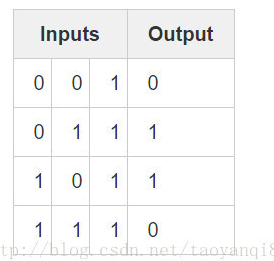Output After Training:[[ 0.5]
[ 0.5]
[ 0.5]
[ 0.5]]1234512345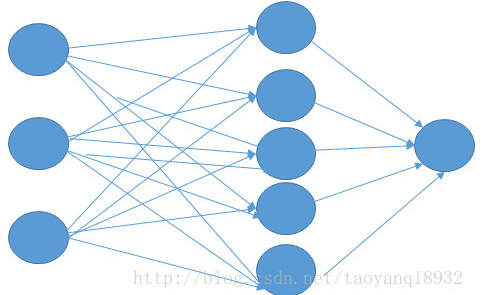import numpy as np
def nonlin(x,deriv=False):
if(deriv==True):
return x*(1-x)
return 1/(1+np.exp(-x))
X = np.array([[0,0,1],
[0,1,1],
[1,0,1],
[1,1,1]])
y = np.array([,
,
,
])
np.random.seed(1)
# randomly initialize our weights with mean 0
syn0 = 2*np.random.random((3,5)) - 1
syn1 = 2*np.random.random((5,1)) - 1
for j in xrange(60000):
# Feed forward through layers 0, 1, and 2
l0 = X
l1 = nonlin(np.dot(l0,syn0))
l2 = nonlin(np.dot(l1,syn1))
# how much did we miss the target value?
l2_error = y - l2
if (j% 10000) == 0:
print "Error:" + str(np.mean(np.abs(l2_error)))
# in what direction is the target value?
# were we really sure? if so, don't change too much.
l2_delta = l2_error*nonlin(l2,deriv=True)
# how much did each l1 value contribute to the l2 error (according to the weights)?
l1_error = l2_delta.dot(syn1.T)
# in what direction is the target l1?
# were we really sure? if so, don't change too much.
l1_delta = l1_error * nonlin(l1,deriv=True)
syn1 += l1.T.dot(l2_delta)
syn0 += l0.T.dot(l1_delta)
print l2

Error:0.500628229093
Error:0.00899024507125
Error:0.0060486255435
Error:0.00482794013965
Error:0.00412270116481
Error:0.00365084766242
# 这一部分是最后的输出结果
[[ 0.00225305]
[ 0.99723356]
[ 0.99635205]
[ 0.00456238]]